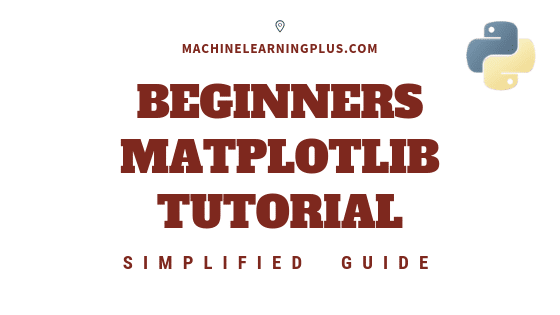## Matplotlib Tutorial – A Complete Guide to Python Plot with Examples

Until next time This tutorial explains matplotlib’s way of making plots in simplified parts so you gain the knowledge and a clear understanding of how to build and modify full featured matplotlib plots. 1. Introduction Matplotlib is the most popular plotting library in python. Using matplotlib, you can create pretty much any type of plot. …